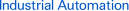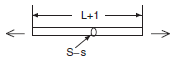Page top# Pressure Sensors / Flow Sensors

With Pressure Sensors, changes in pressure can be measured to confirm suction, verify mounting, manage source pressures, and test for leaking. Differential Pressure Flow Sensors are also available.

 Introduction FeaturesPrinciples Classifications Engineering DataFurther InformationExplanation of Terms Troubleshooting
﻿

What Is a Pressure Sensor?

A pressure sensor is a device equipped with a pressure-sensitive element that measures the pressure of a gas or a liquid against a diaphragm made of stainless steel, silicon, etc., and converts the measured value into an electrical signal as an output.
(The E8[] Pressure Sensors use a silicon diaphragm.)

Features

1. Different sensors are used for different measurement targets, such as liquids, gases, flammable substances, and corrosive substances.
(The E8[] Sensors are used to measure the pressure of non-flammable and non-corrosive gases.)

2. There are sensors that measure the absolute pressure and those that measure the pressure relative to atmospheric pressure or a specified pressure. For sensors that use atmospheric pressure as a reference, there are sensors that measure negative pressures and positive pressures. (The E8Y is a differential pressure sensor. The E8F2 is a gauge pressure sensor that uses atmospheric pressure as the reference.)

Semiconductor Distortion Gauge ConstructionOperating Principles

A semiconductor piezo-resistance dispersion pressure sensor has a semiconductor distortion gauge formed on the surface of the diaphragm, and it converts changes in electrical resistance into an electrical signal by means of the piezo-resistance effect that occurs when the diaphragm is distorted due to an external force (pressure).
Typical model: E8F2

A static capacitance pressure sensor has a capacitor that is formed by a static glass electrode and an opposing movable silicon electrode, and it converts changes in static capacitance that occur when the movable electrode is distorted due to an external force (pressure) into an electrical signal.
Typical model: E8Y

Piezo-resistance EffectThe electrical resistance of the above conductor is expressed by the following formula:
R = px L/S.

p: Electrical resistivity
L: Conductor length
S: Conductor cross-sectional area

When this conductor is pulled to the right or left as shown below, the length increases and the cross-sectional area decreases.The electrical resistance of the above conductor is expressed by the following formula:
R' = px(L+1)/(S-s).

Accordingly,
R' > R.
This shows how the application of a mechanical force changes the electrical resistance.